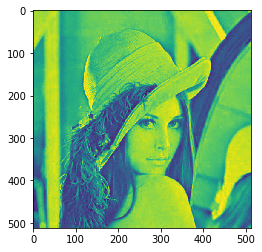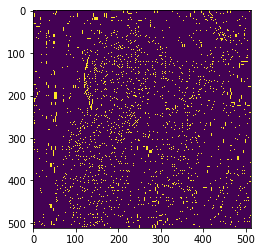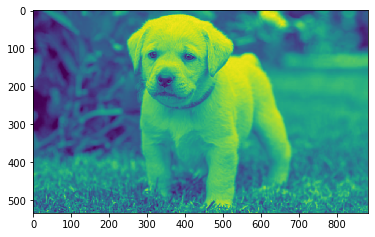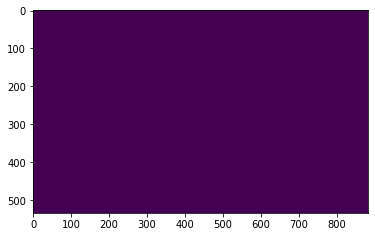GFG App
Open AppBrowser
Continue

# Mahotas – Regional Maxima of Image

In this article, we will see how we can get regional maxima of images in mahotas. Regional maxima is a stricter criterion than the local maxima as it takes the whole object into account and not just the neighborhood. Maxima are connected components of pixels with a constant intensity value, surrounded by pixels with a lower value.

In this tutorial, we will use the “Lena” image, below is the command to load it.

`mahotas.demos.load('lena')`

Below is the Lena imageIn order to do this we will use mahotas.regmax method
Syntax : mahotas.regmax(img)
Argument : It takes image object as argument
Return : It returns image object

Note: Input image should be filtered or should be loaded as grey

In order to filter the image we will take the image object which is numpy.ndarray and filter it with the help of indexing, below is the command to do this

`image = image[:, :, 0]`

Below is the implementation

## Python3

 `# importing required libraries` `import` `mahotas` `import` `mahotas.demos` `from` `pylab ``import` `gray, imshow, show` `import` `numpy as np` `import` `matplotlib.pyplot as plt` `  `  `# loading image` `img ``=` `mahotas.demos.load(``'lena'``)` `  `  `# filtering image` `img ``=` `img.``max``(``2``)`   `print``(``"Image"``)` `  `  `# showing image` `imshow(img)` `show()` `# finding regional maxima` `new_img ``=` `mahotas.regmax(img)` ` `    `# showing image` `print``(``"Regional Maxima"``)` `imshow(new_img)` `show()`

Output :

```Image
````Regional Maxima`Another example

## Python3

 `# importing required libraries` `import` `mahotas` `import` `numpy as np` `from` `pylab ``import` `gray, imshow, show` `import` `os` `import` `matplotlib.pyplot as plt` ` `  `# loading image` `img ``=` `mahotas.imread(``'dog_image.png'``)`     `# filtering image` `img ``=` `img[:, :, ``0``]` `  `  `print``(``"Image"``)` `  `  `# showing image` `imshow(img)` `show()`   `# finding regional maxima` `new_img ``=` `mahotas.regmax(img)` ` `    `# showing image` `print``(``"Regional Maxima"``)` `imshow(new_img)` `show()`

Output :

`Image``Regional Maxima`My Personal Notes arrow_drop_up